Courses

# Test: Design Of Springs (Level - 1)

## 10 Questions MCQ Test Machine Design | Test: Design Of Springs (Level - 1)

Description
This mock test of Test: Design Of Springs (Level - 1) for Mechanical Engineering helps you for every Mechanical Engineering entrance exam. This contains 10 Multiple Choice Questions for Mechanical Engineering Test: Design Of Springs (Level - 1) (mcq) to study with solutions a complete question bank. The solved questions answers in this Test: Design Of Springs (Level - 1) quiz give you a good mix of easy questions and tough questions. Mechanical Engineering students definitely take this Test: Design Of Springs (Level - 1) exercise for a better result in the exam. You can find other Test: Design Of Springs (Level - 1) extra questions, long questions & short questions for Mechanical Engineering on EduRev as well by searching above.
QUESTION: 1

### Two concentric helical springs with spring constants 200 N/mm and 160 N/mm when subjected to a load of 1800 N will deflect by

Solution: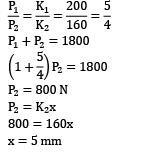QUESTION: 2

### For a helical spring, spring index is

Solution:

For a helical spring, spring index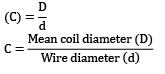QUESTION: 3

### Two spring of stiffness respectively are connected in series, what will be the stiffness of the composite spring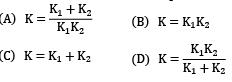Solution:

Let stiffness of the composite spring be kC k1 and k2 are two springs connected in series For series combination,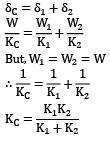QUESTION: 4

Concentric helical springs should be

Solution:

Concentric helical springs are used to provide more spring force in a given space. They should be wound with opposite hand helices to avoid winding up in each other.

QUESTION: 5

If both the mean coil diameter and wire diameter of a helical compression or tension spring be doubled, then the deflection of the spring close coiled under same applied load will

Solution: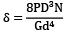QUESTION: 6

Which one of the following statements is correct?If a helical spring is halved in length, its spring stiffness

Solution: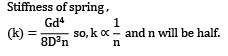QUESTION: 7

A helical compression spring of stiffness k is cut into two pieces, each having equal number of turns and kept side by side under compression. The equivalent spring stiffness of this new arrangement is equal to

Solution:
QUESTION: 8

The equivalent spring stiffness for the system shown in the given figure (S is the spring stiffness of each of the three springs) is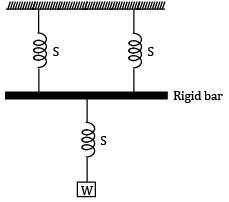Solution: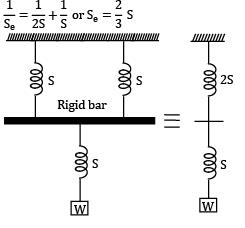QUESTION: 9

In a closed helical spring subjected to an axial load, other quantities remaining the same, if the wire diameter is doubled and mean radius of the coil is also doubled, and then stiffness of spring when compared to original one will become __________.

Solution: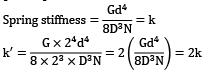QUESTION: 10

Leaf springs are subjected to

Solution:

Leaf springs are used in suspension of automobiles and are subjected to bending stresses.